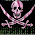## Thursday, 17 January 2013

### Unique Random number generator in vb.net

//hey guys here is my new program in vb.NET which generates unique 20 random Numbers.
Public Class Form1
Dim generator As New Random
Dim arr(20) As Integer
Dim num As Integer = 0
Dim i As Integer = 1
Dim k As Integer = 0
Dim x As Boolean

Public Sub btngenerate_Click(ByVal sender As System.Object, ByVal e As System.EventArgs) Handles btngenerate.Click

numshow()

End Sub

Private Sub numshow()
If k < 20 Then
num = generator.Next(1, 21)
x = check()
If x = True Then

arr(i) = num
Label1.Text = arr(i).ToString
i = i + 1
k = k + 1
Else

numshow()
num = 0
End If

Else
MessageBox.Show("no more")
End If

End Sub
Private Function check() As Boolean
Dim l = i - 1
For j As Integer = 1 To l

If arr(j) = num Then
Return False
End If
Next
Return True

End Function
End Class

If you liked the code please do the courtesy to give one like and follow my blog or please at least visit our youtube channel at least once and subscribe that too if you like.
1.